# 二叉树

##### 什么是树？

• 树是计算机科学中经常用到的一种数据结构
• 树是一种非线性的数据结构，以分层的方式存储数据
• 树被用来存储具有层级关系的数据，比如文件系统中的文件
• 树还被用来存储有序列表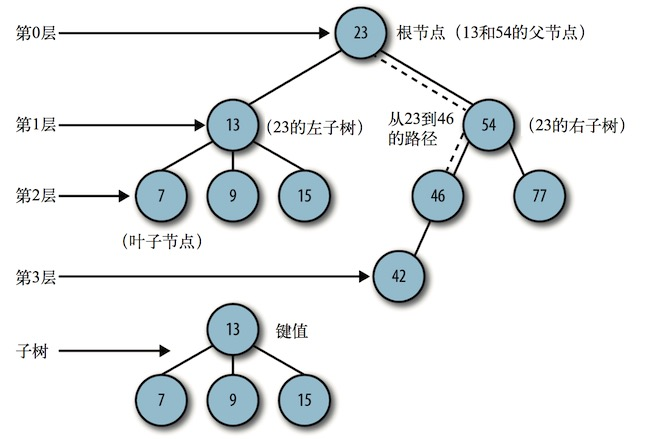• 沿着一组特定的边，可以从一个节点走到另外一个与它不直接相连的节点。从一个节点到另一个节点的这一组边称为路径，在图中用虚线表示。以某种特定顺序访问树中所有的节点称为树的遍历

• 树可以分为几个层次，根节点是第 0 层，它的子节点是第 1 层，子节点的子节点是第 2 层，以此类推。树中任何一层的节点可以都看做是子树的根，该子树包含根节点的子节点，子节点的子节点等。树的层数就是树的深度

• 每个节点都有一个与之相关的值，该值有时被称为

##### 实现二叉查找树
• 二叉查找树由节点组成，所以我们要定义的第一个对象就是 Node，节点

``````function Node(data, left, right) {
this.data = data;
this.left = left;
this.right = right;
this.show = show;
}

function show() {
return this.data;
}
``````
• 再创建一个类，用来表示二叉查找树(BST)

``````function BST() {
this.root = null;
this.insert = insert;
this.inOrder = inOrder;
}
``````
• insert方法：用来向树中加入新节点

(1) 设根节点为当前节点。
(2) 如果待插入节点保存的数据小于当前节点，则设新的当前节点为原节点的左节点;反之，执行第 4 步。
(3) 如果当前节点的左节点为 null，就将新的节点插入这个位置，退出循环;反之，继续执行下一次循环。
(4) 设新的当前节点为原节点的右节点。
(5) 如果当前节点的右节点为 null，就将新的节点插入这个位置，退出循环;反之，继续执行下一次循环。

``````function insert(data) {
var n = new Node(data, null, null);
if (this.root == null) {
this.root = n;
} else {
var current = this.root;
var parent;
while (true) {
parent = current;
if (data < current.data) {
current = current.left;
if (current == null) {
parent.left = n;
break;
}
} else {
current = current.right;
if (current == null) {
parent.right = n;
break;
}
}
}
}
}
``````

• 中序

``````function inOrder(node) {
if (!(node == null)) {
inOrder(node.left);
document.write(node.show() + " ");
inOrder(node.right);
}
}
``````

``````var nums = new BST();
nums.insert(56);
nums.insert(81);
nums.insert(22);
nums.insert(30);
nums.insert(77);
nums.insert(92);
nums.insert(10);
document.write("Inorder traversal: ");
inOrder(nums.root);

``````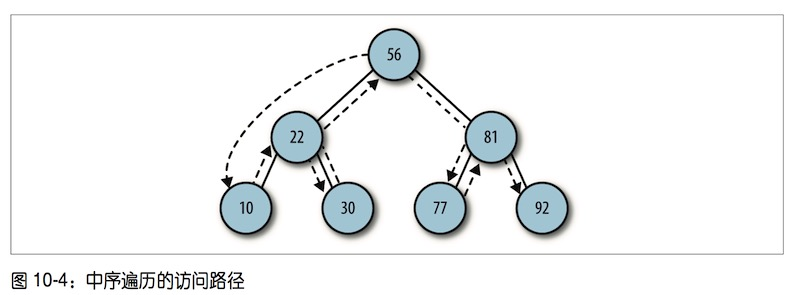• 先序

``````function preOrder(node) {
if (!(node == null)) {
document.write(node.show() + " ");
preOrder(node.left);
preOrder(node.right);
}
}
``````
``````var nums = new BST();
nums.insert(50);
nums.insert(70);
nums.insert(10);
nums.insert(15);
nums.insert(60);
nums.insert(5);
nums.insert(80);
document.write("preOrder traversal: ");
preOrder(nums.root);

``````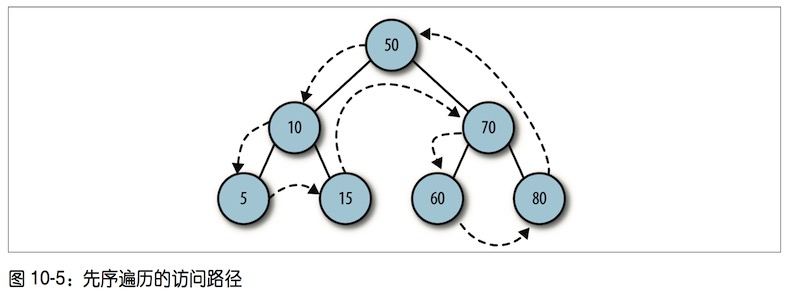inOrder() 和 preOrder() 方法的唯一区别，就是 if 语句中代码的顺序。

(1) 在 inOrder() 方法中，show() 函数像三明治一样夹在两个递归调用之间;
(2) 在 preOrder() 方法中，show() 函数放在两个递归调用之前

• 后序

``````function postOrder(node) {
if (!(node == null)) {
postOrder(node.left);
postOrder(node.right);
document.write(node.show() + " ");
}
}
``````
``````var nums = new BST();
nums.insert(23);
nums.insert(45);
nums.insert(16);
nums.insert(37);
nums.insert(3);
nums.insert(99);
nums.insert(22);
document.write("postOrder traversal: ");
preOrder(nums.root);

``````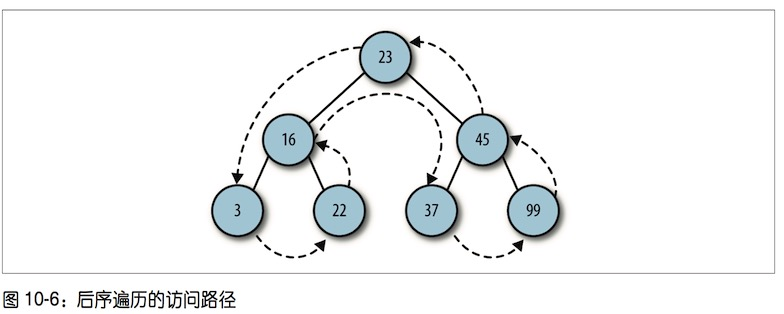##### 在二叉查找树上进行查找

• 查找最小值
``````function getMin() {
var current = this.root;
while (!(current.left == null)) {
current = current.left;
}
return current.data;
}
``````
• 查找最大值
``````function getMax() {
var current = this.root;
while (!(current.right == null)) {
current = current.right;
}
return current.data;
}

``````

``````var nums = new BST();
nums.insert(56);
nums.insert(81);
nums.insert(22);
nums.insert(30);
nums.insert(77);
nums.insert(92);
nums.insert(10);

var min = nums.getMin();
document.write("The minimum value of the BST is: " + min);
document.write("--------");
var max = nums.getMax();
document.write("The maximum value of the BST is: " + max);
``````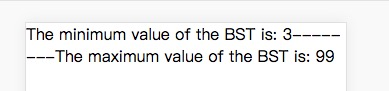• 查找给定值

``````function find(data) {
var current = this.root;
while (current != null) {
if (current.data == data) {
return current;
} else if (data < current.data) {
current = current.left;
} else {
current = current.right;
}
}
return null;
}
``````

##### 从二叉查找树上删除节点

• 从 BST 中删除节点的第一步是判断当前节点是否包含待删除的数据，如果包含，则删除该 节点;如果不包含，则比较当前节点上的数据和待删除的数据。如果待删除数据小于当前 节点上的数据，则移至当前节点的左子节点继续比较;如果删除数据大于当前节点上的数 据，则移至当前节点的右子节点继续比较。
• 如果待删除节点是叶子节点(没有子节点的节点)，那么只需要将从父节点指向它的链接 指向 null。
• 如果待删除节点只包含一个子节点，那么原本指向它的节点久得做些调整，使其指向它的 子节点。
• 如果待删除节点包含两个子节点，正确的做法有两种:要么查找待删除节点左子树 上的最大值，要么查找其右子树上的最小值。这里选择后一种方式

``````function remove(data) {
root = removeNode(this.root, data);
}

function removeNode(node, data) {
if (node == null) {
return null;
}

if (data == node.data) {
// 没有子节点的节点
if (node.left == null && node.right == null) {
return null;
}
// 没有左子节点的节点
if (node.left == null) {
return node.right;
}

// 没有右子节点的节点
if (node.right == null) {
return node.left;
}

// 有两个子节点的节点
var tempNode = getSmallest(node.right);
node.data = tempNode.data;
node.right = removeNode(node.right, tempNode.data); return node;
} else if (data < node.data) {
node.left = removeNode(node.left, data);
return node;
} else {
node.right = removeNode(node.right, data);
return node;
}
}
``````